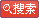# GMATЪ§бЇжаЕФ35ЕРО­ЕфФбЬт

ЗЂВМЪБМфЃК2007-9-3ЁЁЮФзжДѓаЁЃКДѓ жа аЁЁЁДђгЁЃКДђгЁДЫЮФ

1.. вЛУЖгВБвЯђЩЯХзЃЌСНУцЕФЛњТЪИїЮЊвЛАыЃЌЮЪСЌХзШ§ДЮЃЌЭЌвЛУцЕФЛњТЪЁЃЃЈ1/4ЃЉ
ЭЌвЛУцгаСНжжЧщПіЃКЙЪ1/8+1/8=1/4

2. 11ЧђЃЌ6КьЃЌ5РЖЃЌwithout replacement, ЮЪШЁвЛРЖвЛКьЕФpossibility. 6/11
ЕквЛДЮШЁРЖЕФЧщПі C15*C16 .ЕквЛДЮШЁКьЕФЧщПі: C16*C15ЃЌ змЙВЕФЧщПіЮЊC111C110=110 дђИХТЪЮЊ 60/110=6/11

3. Дѓгк700ЕФШ§ЮЛећЪ§жаЃЌгаЖрЩйЪЧЦцЪ§ЃЌвЊЧѓУПИіЪ§зжЖМВЛЮЊСуЃЌЧвУПЮЛЪ§зжЖМВЛЭЌЁЃД№АИЃК 91 How to get the answer?

4. is xy>z? ЮвОѕЕУЪЧC
(1). xyz=1 (2). xyz^2>1 KEY: e why not C? (as NOT is also an answer)

5ЃЎX,Y,ZЪЧШ§НЧаЮЕФШ§ИіБп, ЧвX<Y<Z, Ш§НЧаЮЕФУцЛ§=1, ЮЪYЕФЗЖЮЇ? (Y>2^1/2)
.1/2XYsin(a)=1 Жј1/2XYsin(a)<1/2Y^2sin(a)
ЙЪ1<1/2Y^2sin(a) => Y^2>2/sin(a)>2
Ыљвд Y>2^1/2

6.
O
X X
X X X
X X X X
X X X X X
M M M M
ЩЯЭМЕквЛаа"O"БэвЛаЁЧђ, Ек2,3,4ааЕФ"X"БэеЯА­Юя, Ек6ааБэаЁВл. аЁЧђДгЩЯТфЯТ,дкЕкШ§ааЪБ
ЪмЕНеЯА­ЮяЕФзшжЙ, ЦфЯђзѓЛђгвЕФЛњТЪЯрЕШ, ИїеМ50%. вдЯТвРШЛ. ЮЪзюКѓЧђЕєЕНЕкСљааЕФЕкЖўИі
MЕФЛњТЪ? (3/8)
ЖдУПвЛааТфЕНXЕФИХТЪЖМБэЪОГіРДЃЌШЛКѓМДПЩЕУГіНсТлЮЊ3/8

7. TWO KEYSЃЌ ЗХЕНвбга5АбдПГзchainжаЃЌЮЪетСНАбдПГзЯрСкЕФИХТЪ ЃП
NO.3 гІИУЪЧЛЗаЮЕФ. ЩЯУцД№АИЪЧЛњОЎЩЯЕФ. ЮвЕФД№АИЪЧ5/P2,10 = 1/9 ВЛжЊЖдТ№?дкЛЗаЮCHAINЩЯ, ЙВгаЮхИіПеЮЛ, УПИіПЩЗХвЛИіЛђЖўИіKEY, ПЩЕУP2,10
ЯрСйЧщПіЮЊЮхИі.ЕУЕН1/9

8.ИјГіБъзМЗНВюЙЋЪНЃЌгавЛзщЪ§1ЃЌ3ЃЌ5ЃЌ7ЁЃЁЃЁЃ19 ЃЌЯждкБфЖЏвЛЯТЃЌЮЪаТБэзМЗНВюAЃЌBЃЌCЃЌDЃЌEЕФMEANЪЧ16ЃЌEЮЊ40ЃЌЧвE>D>C>B>AЃЌ ЧѓCЕФзюДѓПЩФмжЕЃП
БъзМВюЕФЙЋЪНЮЊ:
sqrt((a1-a)^2+(a2-a)^2+...+(an-a)^2) Цфжаa=(a1+a2+..+an)/nЃЌЁЃдѕУДБфЖЏЃПФуУЛЫЕАЁ

9.ФГВЭЬќга2жжВЛЭЌЫЎЙћ, 6жжВЛЭЌЕАИт, ШєВЭКѓЬ№ЦЗУПДЮЖМвдa same number of kinds of fruit and cake, дђИУВЭЬќгаМИжжВЭКѓЬ№Еу? ИљОнЮвЕФРэНт, C2,1C6,1+C2,2C6,2=12+15=27ЁЃ27ЮЊбЁЯюEЁЃбЁЯюBЮЊ12ЁЃ

10. ФГИіЙЋЫОжАдБДѓгк10ШЫаЁгк40ШЫ, ПЊЛсЪБШєУП4ШЫгУвЛИізРзг, гр3ШЫЖРгУвЛИі; ШєУП5ШЫгУвЛИі, дђгр3ШЫЖРгУвЛИі. ЮЪЯж6ШЫгУвЛИізРзг, НЋгрМИИіЖРгУвЛИізРзг.
етЬтБШНЯМђЕЅЁЃ етУДПМТЧЃЌШЫЪ§БЛ4Г§гр3ЃЌБЛ5Г§гр3ЃЌЮЪБЛ6Г§грМИЃП
10<4a+3<40 10<5b+3<40 дђДЫЪ§ЮЊ: 23 дђБЛ6Г§гр5

11. If x and y are positive integers such that x=8y+12,what is the greatest common divisor of x and y?
1) x=12u,where u is an integer
2) y=12z,where z is НтД№ЃКгЩЬтжЊ: x=4(2y+3)
гЩ(1)жЊ: x=12u дђy=3k k is an integer. x=12(2k+1) дђx,yзюДѓЙЋвђзгВЛФмШЗЖЈ.
гЩ(2)жЊ: y=12z дђx=12(6z+1) ,вђДЫx,yЕФЙЋвђзгЪЧ12ЁЃ
етЬтЮвШЯЮЊбЁBan integer

12. 2^100-22^96, ЮЪЦфзюДѓЕФжЪвђзгЪЧМИ?(Д№АИЮЊ5)
2^100-22^96,ПЯЖЈЪЧЬтФПгаЮѓЃЌПЩФмЪЧ2^100-2^96. 2^96(2^4-1)------2^96*3*5, вђДЫЮЊ5ЁЃ

13. The possibility that the value of Stock A will increase is 0.34 and the possibility that stock B will increase is0.68 What is the biggest possibility that neither will happen? (I am not sure about the numbers.)
I am not very sure if I was correct for this question, therefore I prefer not to mislead you. However, the answer is absolutely not (1-0.34)*(1-0.68).
ИХТЪP=ТњзуФГИіЬѕМўЕФЫљгаПЩФмЧщПіЪ§СП/ЫљгаПЩФмЧщПіЪ§СП
аджЪ 0<=P<=1
a1,a2ЮЊСНСНВЛЯрШнЕФЪТМўЃЈМДЗЂЩњСЫa1ЃЌОЭВЛЛсЗЂЩњa2)
P(a1Лђa2)=P(a1)+P(a2)
a1,a2ВЛЪЧСНСНВЛЯрШнЕФЪТМўЃЌЗжБ№гУМЏКЯAКЭМЏКЯBРДБэЪО
МДМЏКЯAгыМЏКЯBгаНЛМЏЃЌБэЪОЮЊA*B ЃЈa1ЗЂЩњЧвa2ЗЂЩњЃЉ
МЏКЯAгыМЏКЯBЕФВЂМЏЃЌБэЪОЮЊA U B ЃЈa1ЗЂЩњЛђa2ЗЂЩњЃЉ
дђ
PЃЈA U BЃЉ= P(A)+P(B)-P(A*B)ЁЃЁЃЁЃЁЃЁЃЁЃЁЃЁЃЁЃЁЃЁЃЁЃЁЃЁЃЁЃЁЃЁЃЙЋЪН2
ЛЙгаОЭЪЧЬѕМўИХТЪЃК
ПМТЧЕФЪЧЪТМўAвбЗЂЩњЕФЬѕМўЯТЪТМўBЗЂЩњЕФИХТЪ
ЖЈвхЃКЩшAЃЌBЪЧСНИіЪТМўЃЌЧвPЃЈAЃЉ>0,ГЦ
PЃЈB|AЃЉ=PЃЈA*BЃЉ/PЃЈAЃЉ

14. XЕФИїЮЛКЭЪЧ170. X=20^17-Y,ЮЪY. ПЩбЁЯюЪЧДг53ЕН6?
етИіЬтгааЉРрЫЦФЧЕРМѕШЅСНЮЛЪ§ЕФЬтЁЃx=10^17*2^17-y=131072*10^17-y
170-13=157
гааЉЮЪЬт,ШчЙћЪЧ157ЕФЛАЃЌетбљФЉ17ЮЛЖМЪЧ9ЕФЛАвВВЛЙЛ157.дѕУДЛиЪТЃП зіЗЈПЯЖЈЪЧетУДзіЕФЃЁ

15. R,T,UдкдВЩЯ,АыОЖЮЊ4,ЛЁRTU=4/3PAI, ЮЪRUЕФSEGMENT LENTH
НЧROU=360X(ЛЁГЄ/жмГЄ)=360X(4/3PI/8PI)=60ЃЌвђДЫRU= r =4

16. nЮЊ1~100 (КЌ)МфећЪ§ЃЌЮЪn(n+1) ФмБЛ4ећГ§ЕФИХТЪЁЃ
n(n+1)ПЯЖЈФмБЛ2ећГ§.ШчЙћn(n+1)ФмБЛ4ећГ§ЃЌФЧУДnЛђепn+1ФмБЛ4ећГ§ЃЌдк1~100жаnФмБЛ4ећГ§ЕФга25Иі.n+1ФмБЛ4ећГ§ЕФга25ИіЃЌвђДЫ ИХТЪЮЊ (25+25)/100=1/2
3 s/tЃН64.12=6412/100 .етИіЬтГ§вЛЯТЗЂЯжНсТлШчЯТ: 1603=64*25+3 , дђгрЪ§ПЯЖЈФмБЛ3ећГ§ЃЌвђДЫжЛФмбЁ45. E

17. 1. 0<x<1,which is right?
ЦфжагавЛИіЪЧx^4-x^5<x^2-x^3,Д№АИЪЧЖдЕФЁЃЮвВЛжЊЕРИУдѕУДХаЖЯЁЃ
x^4-x^5<x^2-x^3 => x^4(1-x)<x^2(1-x)
вђЮЊ 0<x<1 Ыљвд 1-x>0 ЫљвдЩЯЪНЮЊ x^4<x^2 ЃЌетИівђЮЊ 0<x<1ЃЌЫљвдГЩСЂЁЃ

18ЃЎA certain musical scale has 13 kinds.УПжжРраЭгаВЛЭЌЕФЦЕТЪЁЃДгаЁЕНДѓХХСаЃЌзюДѓЕФЦЕТЪЪЧзюаЁЕФ2БЖЁЃГ§зюДѓЕФЭтЃЌЪЃгрЕФ12ИіГЩЕШБШХХСаЁЃШєзюаЁЕФЦЕТЪЪЧ440ЃЌдђЕк7ИіЪЧЖрЩйЁЃ
Д№АИЮЊ440*ИљКХ2ЁЃЭъШЋВЛжЊЕРИУдѕУДзіЁЃ
ЩшЕШБШЮЊq Цфжаq>1,вђЮЊДгаЁЕНДѓХХСа. га a13=2a1 a7=a1*q^6
Жјa12=a1*q^11 га a1*q^11<2a1 дђ q^11<2
етИіЬтЛсВЛЛсВЛШЋЃП

19.ГЄЗНаЮЃЌжмГЄPЃЌУцЛ§KЃЌФФИівЛЖЈЖдЃП
Д№АИЪЧ2w^2-pw+2k=0.ЭъШЋВЛжЊЕРИУдѕУДзіЁЃ
ПэW,жмГЄP,УцЛ§K ,дђ ГЄ=K/W жмГЄ=2(K/W+W)=P
Ыљвдга2w^2-pw+2k=0

20ЃЎIf x and y are positive integers such that x=8y+12,what is the greatest common divisor of x and y?
1) x=12u,where u is an integer
2) y=12z,where z is an integer
the key is D. How to deal with this kind of question.
гЩЬтжЊ: x=4(2y+3)
гЩ(1)жЊ: x=12u дђy=3k k is an integer. x=12(2k+1) дђx,yзюДѓЙЋвђзгВЛФмШЗЖЈ.
гЩ(2)жЊ: y=12z дђx=12(6z+1) ,вђДЫx,yЕФЙЋвђзгЪЧ12ЁЃ
етЬтЮвШЯЮЊбЁB

21. If xyВЛЕШгк0,is x/y ЕШгк0Т№ЃП
1ЃЉ x=-y 2)-x=y
Д№АИЪЧDЁЃЕЋЮвИаОѕ1ЃЉЃЌ2ЃЉетСНИіЬѕМўКУЯѓУЛгагУЁЃ
гЩxyЁй0 => xЁй0 Чв yЁй0, вђДЫx/yПЯЖЈВЛЕШгк0ЁЃЕЋЪЧЪ§ОнГфЗжадЕФЬтЃЌФуВЛвЊЙмЫќга
УЛгагУЃЌвтЫМЪЧЫЕШчЙћ(1)ГЩСЂЃЌФуФмЭЦГіНсТлТ№ЃПШчЙћФмЃЌОЭok.ЫљвдбЁD

22ЃЎOn JaneЁЏs credit card account, the average daily balance for a 30-day billing cycle is the average of the daily balances at the end of each of the 30 days.At the beginning of a certain 30-day billing cycle,JaneЁЏs credit card account had a balance of \$600.Jane made a payment of \$300 on the account during the billing cycle.If no other amounts were added to or subtracted from the account during the billing cycle, what was the average daily balance on JaneЁЏs account for the billing cycle?
1) JaneЁЏs payment was credited on the 21st day of the billing cycle.
2) The average daily balance through the 25th day of the billing cycle was \$540.
The key is D,but why?
ПМдФЖСЕФЬт,ЮвЖСЕФКмРлЃЌФмВЛФмЗ­выГЩжаЮФ:(

23. 1Иіе§ЗНаЮЗжГЩвЛаЉаЁИёзгЃЈИёзгзмЪ§ФПвбжЊЃЉЃЌУПИіИёзгРягавЛИіЪ§зжЃЌЧѓЫљгаЪ§зжЕФЦНОљЪ§ 1 вбжЊИїКсЯђЦНОљЪ§ЕФзмКЭ 2 вбжЊИїзнЯђЦНОљЪ§ЕФзмКЭСюИёзгзмЪ§ЮЊ m*n ЦфжаmЮЊааЪ§, nЮЊСаЪ§
вбжЊИїКсЯђЕФЦНОљЪ§змКЭЃЌБэЪО.ЫљгаЪ§зжКЭЮЊ n(s1+s2+..+sm) дђЦНОљЪ§ЮЊ
n(s1+s2+..+sm)/(m*n)=(s1+s2+..+sm)/m ,ЖјmЕФжЕД§ЖЈЃЌЫљвдЦНОљЪ§ЧѓВЛГі. ЖјзмКЭ(1) (2)ЪЧПЩвдАбНсЙћЧѓГіРДЕФЃЌНсЙћгыm,nЮоЙи

24. гаЭМЫЕЪЧвЛИізЊХЬЃЌЗжЮЊ8ИіЧјгђЃЌЗжБ№ДњБэ1ЕН8ЃЌМ§ЭЗзЊЕНФФИіЧјгђЃЌФЧИіШЫОЭзпЖрЩйВНЃЌЃЈКмЯёжаЙњЕФвЛжжгЮЯЗЃЉ
ЮЪвЛЙВ3ДЮзЊвЊзп16ВНЕФЛАЃЌгаЖрЩйжжВЛЭЌЕФХХСаЫГађЁЃЃЈетЕНЬтЮвЫуСЫКУОУЃЌзюКѓЛЙЪЧВЛШЗЖЈЃЌЕквЛДЮЪ§бЇАбЮвзїЕФетУДгєУЦЃЉ
Д№АИдк56 КЭ64жЎМфХЧЛВАЩЁЃ
[ зЊЛЏГЩПЩвджиИДЕФДг1-8жаШЮвтбЁШ§ИіЪ§ЃЌКЭЮЊ16ЕФХХСаЪ§ЮЊЖрЩйЃПЯШАбЫљгаЕФПЩФмзщКЯевГіРДдйХХСа
га1ЕФЧщПі 1,7,8
га2 ЕФЧщПі 2, 6, 8 2,7,7
га3ЕФЧщПі 3,5,8 3,6,7
га4ЕФЧщПі 4,4,8 4,5,7 4,6,6
га5ЕФЧщПі 5,5,6 (ЮхЕФЦфЫќЧщПігыЧАУцЕФЖМжиИД)
гЩгкХХСаЕФЙиЯЕЃЌЙ§АыЪ§вдКѓГіЯжЕФзщКЯгыЧАУцЕФБиШЛжиИД
ЧАУцЕФЙВга5жжШ§ИіЪ§зжВЛЭЌЕФзщКЯХХСаЪ§=5XP3,3=30ЃЌ4жжСНИіЪ§вЛбљЕФзщКЯХХСаЪ§=4XC3,1=12
вђДЫНсЙћгІИУ=30+12=42]

25..
вЛЕРМИКЮЬтЃЌУшЪіШчЯТЃЌДѓаЁСНдВдкДѓдВзѓВрФкЧаЃЌДЉЙ§СНдВаФBЁЂDМАЧаЕуAЕФжБЯпНЛДѓдВгкEЁЂНЛаЁдВгкCЃЌЮЪДѓдВФкЁЂаЁдВЭтЕФУцЛ§ЃП
A. CD=1ЁЂDE=5
B. BC=2ЁЂCD=1
key:D
ВЛжЊCдкДѓдАаФЕФзѓБпЛђгвБпЃЌЮвCHOOSE E

26.10 pЗНЗЈгыrЗНЗЈЯпадЙиЯЕЃЌ P(6)->R(30) P(24)->R(60) ЧѓR=100ЪБЃЌ p=?
[ key 48 ]
Сюp=ar+b
ШЛКѓДњШыСНИіЪ§ПЩвдАбa,bЫуГіРДЃЌзюКѓАбr=100ДњНјШЅЕУНсТлЃЁ

27. вЛИіТжХЬЗжАЫПщЃЌШ§ДЮзЊеыжИЯђЪ§КЭЮЊ16ЕФsequenceЪ§ФПЃП ЦфЪЕОЭЪЧвдЧАЕФЭЖЗЩякЮЪЬтЁЃЃЈ42ЃЉ
етИіЬтКЭФЧИіШгЁАЪВУДЖЋЮїЁБЃЌШЛКѓЕУГі16ЪЧЭЌвЛЕРЬтЃЌетЕРЬтЕФНтЗЈЪЧУЖОй.
ЕквЛДЮШчЙћЪЧ8,дђга7жжЧщПі
ЕквЛДЮШчЙћЪЧ7,дђга8жжЧщПі
ЕквЛДЮШчЙћЪЧ6,дђга7жжЧщПі
ЕквЛДЮШчЙћЪЧ5,дђга6жжЧщПі
..
ЕквЛДЮШчЙћЪЧ1,дђга2жжЧщПі
змЙВЮЊ 7+8+7+6+...+2=15+(2+7)*6/2=15+27=42

28. гаШ§ИіСЌајећЪ§r s t ЃЌгавЛБэДяЪН N=3^r+3^s+3^t,ЮЪдквдЯТбЁЯюжазюДѓЕФвђзгЪЧЖрЩйЃПбЁЯюга3ЃЌ7ЃЌ13ЃЌ17ЕШЁЃ13
Сюr=1 s=2 t=3 n=3(1+3+9)=3*13 ЛЙгаЃЌгІИУЪЧжЪвђзгАЩ

29. 3ЕФXДЮЗН+1ФмБЛ10ећГ§Т№ЃП C
1)ЪЎЮЛЪЧИіЮЛЕФвђзг 2)ИіЮЛЪЧ2
3^xЕФИіЮЛЕФПЩФмадЮЊ:3,9,7,1
1)ЪЎЮЛЪЧИіЮЛЕФвђзгВЛФмБэЪОЪВУДЃЌ1)ЭЦВЛГі. 2)ЮвОѕЕУИќЭЦВЛГі.ЫљвдD ЃКyes no question

30. гавЛЕРJJЃЌA1--A4б­ЛЗЃЌЧѓЧА97ЯюжЎКЭЁЃЃЈ74ЃЉ
ЯпnЪмЗёБШЯпpаБТЪДѓЃП
ЃЈ1ЃЉСНЯпОљЙ§ЃЈ5ЃЌ1ЃЉ
ЃЈ2ЃЉЯпnЕФyжсНиОрБШЯпpДѓЁЃ
гавЛЕРЗбЗбНВвхЩЯЕФЬтЃЌвЛИіИДКЯКЏЪ§ЕФЭМЯёЃЌзюКѓД№АИЪЧ(Y=X^3-X)
вЛЕРЬтПМВьШЁећКЏЪ§ЕФЖЈвхЃК[x]=-1,ЮЪxЕФЗЖЮЇЁЃЃЈ-1<=x<0ЃЉ

31. The three digits number is K, what is the number of tenths of K?
a. the sum of tenths of K plus 9 is 3.
b. the sum of tenths of K plus 4 is 2.
етЬтЪЧC, злКЯ(1)(2)ПЩвдЕУГіЪЎЮЛЪЧ2

32. X ЁЖ YЃЌ XЃЌYЖМЪЧШ§ЮЛЪ§ЁЃXЕФЪЎЗжЮЛЪЧ5ЃЌYЕФЪЎЗжЮЛЪЧ7ЃЌЯШдкX+Y ЃЌЮЪФФвЛИіMUST BE TRUE
I XЃЌYИїздЕФИіЮЛЪ§аЁгкКЭЕФИіЮЛЪ§
II XЃЌY ЕФКЭЕФЪЎЗжЮЛЪЧ2
IIIЃЌYЕФАйЗжЮЛAT LEAST 5
[ ЬтФПгІИУВЛШЋЃЌАДФПЧАЬѕМўIЃЌIIЃЌIIIЖМВЛФмБЃжЄMUSTЁЁBEЁЁTRUE ]
етЬтЮвУўpp3ЕФЪБКђКУЯѓзіЙ§.етИіЬтЕФвтЫМЪЧПМТЧНјЮЛвдМАЪ§ЕФДѓаЁКЭЫќЗЧЪзЮЛЕФБ№ЕФЪ§зжЕФДѓаЁЮоЙи.
aМйШчx=250 y=370 дђIНсТлДэ
bМйШчx=259 y=379 дђIIНсТлДэ
cМйШчx=150 y=370 дђIIIДэЁЃ
вђДЫI,II,IIЖМВЛФмБЃжЄmust be true.

33. nИіСЌајећЪ§ЕФЛ§зюКѓ6ИіЮВЪ§ЮЊ0ЃЌЮЪnжСЩйЮЊЖрЩй
A 20
B 25
C 30
D 35
Д№АИОЭЪЧ25
етИіЬтЮвД№Й§.2ВЛгУПМТЧЃЌжЛгУПМТЧ5ЃЌn!жага5,10,15,25ОЭга5^6СЫЃЌЫљвдД№АИЪЧ25

34. [(400^300+200^600)/2]^4=400^k,ЮЪK=ЃП
K=1200 ЃЌзіЗЈЃК [(400^300+200^600)/2]^4=400^k
[(400^300+400^300)/2]^4=(400^1200+400^1200)*1/2=400^1200(1+1)*1/2=400^1200
ЭЦГі 400^1200=400^k k =1200

35. is xy>z?
(1). xyz=1 (2). xyz^2>1
1ЃЉx=1/2ЃЌy=1ЃЌz=2ЃЌдђxyЁДzЃЛx=1/2ЃЌy=2ЃЌz=1ЃЌдђxy=z -------AЕЅЖРВЛаа
2ЃЉШєz=5ЃЌxy=1ЃЌдђxyЁДZЃЛ z=1/2ЃЌxy=100ЃЌдђxyЁЕz------BЕЅЖРвВВЛаа
3ЃЉСНепзлКЯЃЌПЩЕУxyЁЕ0 вдМА ZЁЗ1ЃЌдђX*YБиаЁгк1ЃЌЙЪД№АИЮЊЃКC

ЮФеТЃКЁАGMATЪ§бЇжаЕФ35ЕРО­ЕфФбЬтЁБе§ЮФЭъ
• ЯТвЛЦЊЮФеТЃК
• ШШЕуЮФеТ
дкЯпзЩбЏ
ЁЁ